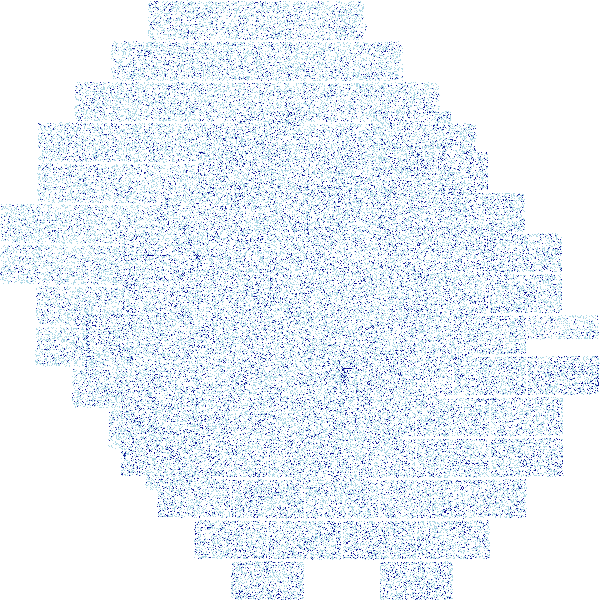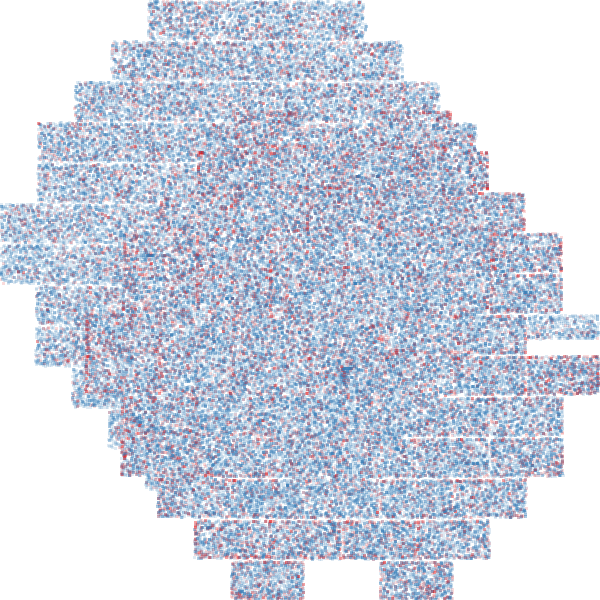# An interactive SMASH density map¶

## Visualization¶

In this notebook, we will use Datashader and Bokeh to make an interactive density map of SMASH all-sky photometry.

## Known issues¶

With this prototype notebook, there is not yet a way to clearly identify the field numbers or coordinates in the all sky plot.

### Initialization¶

We need modules from the Bokeh library, Datashader, NumPy, Pandas, and pyproj, as well as the Data Lab modules to connect to the database.

In :
print "Start"
import bokeh.plotting as bp
from pyproj import Proj
import numpy as np

from cStringIO import StringIO
from dl import authClient
from dl import queryClient

import pandas as pd

# Get the security token for the datalab demo user
print "Got token",token

Start
Got token anonymous.0.0.anon_access


### Querying the SMASH DR1 catalog¶

We will query the entire SMASH catalog, but with a somewhat restricted sharp, magnitude, and color range, as well as a limit on the depth flag, to limit the number of objects returned.

In :
%%time
depth = 1                   # minimum depth
raname = 'ra'
decname = 'dec'
mags = 'gmag,rmag'
dbase='smash_dr1.object'

# Create the query string.
query = ('select fieldid,'+raname+','+decname+','+mags+',depthflag from '+dbase+ \
' where (depthflag > %d and ' + \
' (abs(sharp) < 0.5) and ' + \
' (gmag is not null) and (rmag is not null) and' + \
' (gmag between 18 and 24) and ' + \
' ((gmag-rmag) between -1 and 3))') % \
(depth)

print "Making query"

# Call the Query Manager Service
response = queryClient.query(token, adql = query, fmt = 'csv')

print len(df), "objects found."

Your query is: select fieldid,ra,dec,gmag,rmag,depthflag from smash_dr1.object where (depthflag > 1 and  (abs(sharp) < 0.5) and  (gmag is not null) and (rmag is not null) and (gmag between 18 and 24) and  ((gmag-rmag) between -1 and 3))
Making query
5445976 objects found.
CPU times: user 4.27 s, sys: 875 ms, total: 5.15 s
Wall time: 1min 1s


### Data munging¶

We'll use our Pandas DataFrame to add a blue/red category for objects with colors bluer/redder than g-r=0.4, and use pyproj to project the coordinates to a Goode Homolosine projection.

In :
p1=Proj(proj='goode')
df["g_r"]=df["gmag"]-df["rmag"]
lat=np.array(df["dec"])
lon=np.array(df["ra"]-180)
x,y=p1(lon,lat)
df["x"]=x/1e6
df["y"]=y/1e6

colthresh=0.4  #g-r>0.4 == Red
df["Class"]='Blue'
df.loc[df["g_r"]>colthresh,"Class"]='Red'
df["Class"]=df["Class"].astype('category')
df.tail()

Out:
fieldid ra dec gmag rmag depthflag g_r x y Class
5445971 20 35.705222 -66.790792 23.9966 23.9193 2 0.0773 -8.034689 -7.163028 Blue
5445972 20 35.705774 -66.789700 23.8267 23.5245 2 0.3022 -8.034889 -7.162933 Blue
5445973 20 35.696410 -66.784513 23.4505 23.3245 2 0.1260 -8.036501 -7.162478 Blue
5445974 20 35.699459 -66.783013 23.4188 23.2759 2 0.1429 -8.036647 -7.162346 Blue
5445975 20 35.669377 -66.779954 23.6111 23.0070 2 0.6041 -8.038966 -7.162078 Red

### A density map for Field 169¶

Datashader creates a canvas from the two-dimensional data that you select, aggregates the data, and then uses a transfer function to map the aggregated data onto the canvas. In one fell swoop, limited to Field 169:

In :
df2=df[df["fieldid"]==169]

Out:### Field 169 density map colored by category¶

Here we break down Datashader's steps and a couple of more parameters, such as specifying a log stretch in the transfer function and use our Blue/Red category in making the aggregate. We'll also spread the points out a bit to make theam easier to see. There are many more parameters decribed in the Datashader documentation.

In :
canvas = ds.Canvas(plot_width=600, plot_height=600)
agg  = canvas.points(df2, 'ra', 'dec', ds.count_cat("Class"))

/dl1/sw/anaconda2/lib/python2.7/site-packages/datashader/transfer_functions.py:258: RuntimeWarning: invalid value encountered in true_divide
r = (data.dot(rs)/total).astype(np.uint8)
/dl1/sw/anaconda2/lib/python2.7/site-packages/datashader/transfer_functions.py:259: RuntimeWarning: invalid value encountered in true_divide
g = (data.dot(gs)/total).astype(np.uint8)
/dl1/sw/anaconda2/lib/python2.7/site-packages/datashader/transfer_functions.py:260: RuntimeWarning: invalid value encountered in true_divide
b = (data.dot(bs)/total).astype(np.uint8)

Out:### The interactive all-sky density map¶

We can combine Datashader with Bokeh's interactive plotting to make a dynamic density map of our entire SMASH query result. The code below makes use of Datashader's dynamic spread transfer function, which gives the map variable resolution as a function of zoom level. Try the Wheel Zoom function or Box Zoom to zoom in on a particular field. The field at (-4,-7.5) has a couple of background galaxy clusters in it.

In :
bp.output_notebook()
p = bp.figure(tools='pan,wheel_zoom,box_zoom,reset',x_range=(-10,10), y_range=(-10,0))

def image_callback(x_range, y_range, w, h):
cvs = ds.Canvas(plot_width=w, plot_height=h, x_range=x_range, y_range=y_range)
agg = cvs.points(df, 'x', 'y', ds.count_cat("Class"))